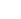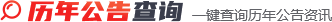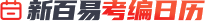2023年11月27日~12月03日MORE+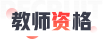MORE+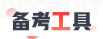MORE+MORE+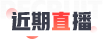MORE+MORE+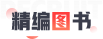MORE+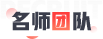MORE+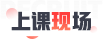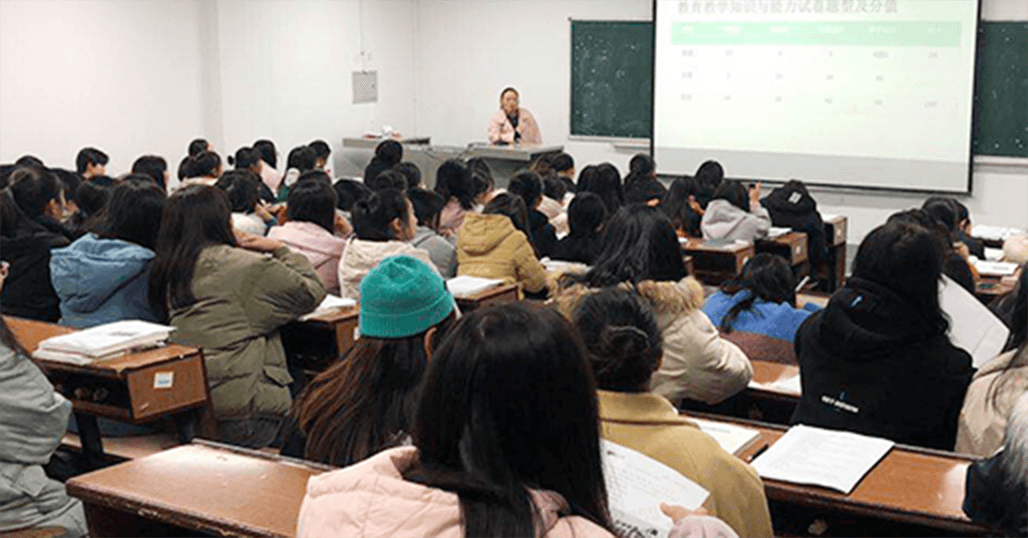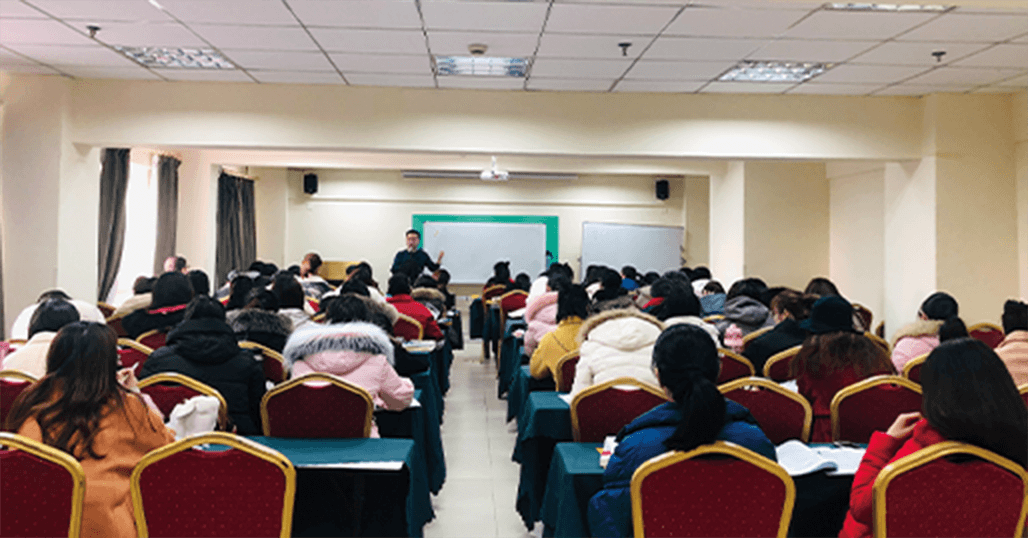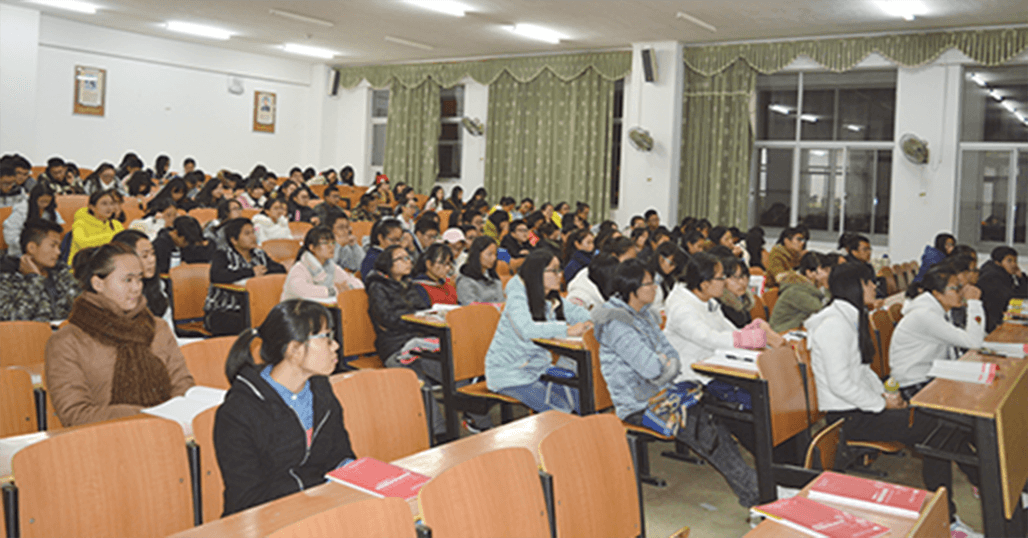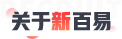MORE+

700 万+23600 课时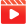30000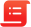MORE+© 2023 Baidu - GS(2023)3206号 - 甲测资字11111342 - 京ICP证030173号 - Data © 百度智图
#
R
G
B
H
S
B
OK
#
R
G
B
H
S
B
OK
#
R
G
B
H
S
B
OK
#
R
G
B
H
S
B
OK
#
R
G
B
H
S
B
OK
#
R
G
B
H
S
B
OK
#
R
G
B
H
S
B
OK
#
R
G
B
H
S
B
OK
#
R
G
B
H
S
B
OK
#
R
G
B
H
S
B
OK
#
R
G
B
H
S
B
OK
#
R
G
B
H
S
B
OK
#
R
G
B
H
S
B
OK
#
R
G
B
H
S
B
OK
#
R
G
B
H
S
B
OK
#
R
G
B
H
S
B
OK
#
R
G
B
H
S
B
OK
#
R
G
B
H
S
B
OK
#
R
G
B
H
S
B
OK
#
R
G
B
H
S
B
OK
#
R
G
B
H
S
B
OK
#
R
G
B
H
S
B
OK
#
R
G
B
H
S
B
OK
#
R
G
B
H
S
B
OK
#
R
G
B
H
S
B
OK
#
R
G
B
H
S
B
OK
#
R
G
B
H
S
B
OK
#
R
G
B
H
S
B
OK
#
R
G
B
H
S
B
OK
#
R
G
B
H
S
B
OK
#
R
G
B
H
S
B
OK
#
R
G
B
H
S
B
OK
#
R
G
B
H
S
B
OK
#
R
G
B
H
S
B
OK
#
R
G
B
H
S
B
OK
#
R
G
B
H
S
B
OK
#
R
G
B
H
S
B
OK
#
R
G
B
H
S
B
OK
#
R
G
B
H
S
B
OK
#
R
G
B
H
S
B
OK
#
R
G
B
H
S
B
OK
#
R
G
B
H
S
B
OK
#
R
G
B
H
S
B
OK
#
R
G
B
H
S
B
OK
#
R
G
B
H
S
B
OK
#
R
G
B
H
S
B
OK
#
R
G
B
H
S
B
OK
#
R
G
B
H
S
B
OK
#
R
G
B
H
S
B
OK
#
R
G
B
H
S
B
OK
#
R
G
B
H
S
B
OK
#
R
G
B
H
S
B
OK
#
R
G
B
H
S
B
OK
#
R
G
B
H
S
B
OK
#
R
G
B
H
S
B
OK
#
R
G
B
H
S
B
OK
#
R
G
B
H
S
B
OK
#
R
G
B
H
S
B
OK
#
R
G
B
H
S
B
OK
#
R
G
B
H
S
B
OK
#
R
G
B
H
S
B
OK
#
R
G
B
H
S
B
OK
#
R
G
B
H
S
B
OK
#
R
G
B
H
S
B
OK
#
R
G
B
H
S
B
OK
#
R
G
B
H
S
B
OK
#
R
G
B
H
S
B
OK
#
R
G
B
H
S
B
OK
#
R
G
B
H
S
B
OK
#
R
G
B
H
S
B
OK
#
R
G
B
H
S
B
OK
#
R
G
B
H
S
B
OK
#
R
G
B
H
S
B
OK
#
R
G
B
H
S
B
OK